# Target Xbar-s Charts

Target Xbar and s (Xbar-s) charts can help you identify changes in the average and standard deviation of a characteristic. Review the following example—an excerpt from Innovative Control Charting1—to get a sense of how a target Xbar-chart works.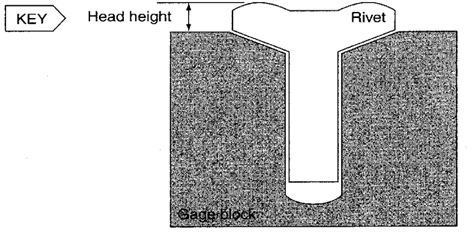Figure 1. Rivet head height is a key characteristic. The measurement is taken with the aid of a gauge block.

## Case Description

Rivet head height is a key characteristic. The height is measured off a gauge block. If the height is too low, the installed rivet will recede below the surface. If it is too high, it will protrude. Either case requires rework and is unacceptable. Three different types of rivets are manufactured, each with different target head heights and tolerances.

Table 1. Target head heights and specifications.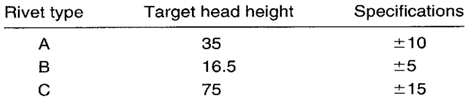## Sampling Strategy

Several rivet types are to be plotted on the same chart, but because only one characteristic, head height, is to be controlled, use of a target chart would be appropriate. The production volume is extremely high (thousands per hour), the data collection is quick, and the analysis is being done with the assistance of computer software. For all these reasons, a target Xbar-s chart is selected.

To determine how often measurements should be taken, a header mechanic is surveyed. It is revealed that adjustments to the equipment affecting head height are made about every hour. To capture the effects of these adjustments, samples of 10 are taken every 10 minutes.

## Data Collection Sheet

Table 2. Data collection sheet for three different rivet head heights.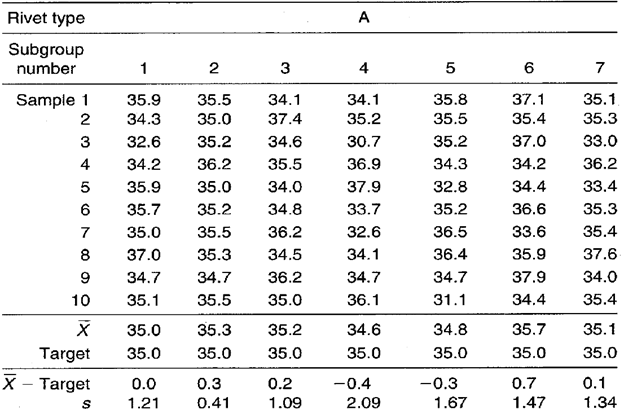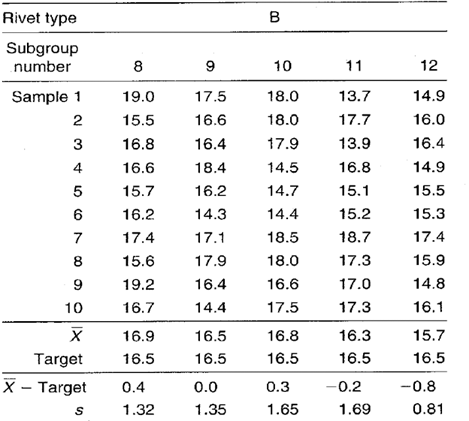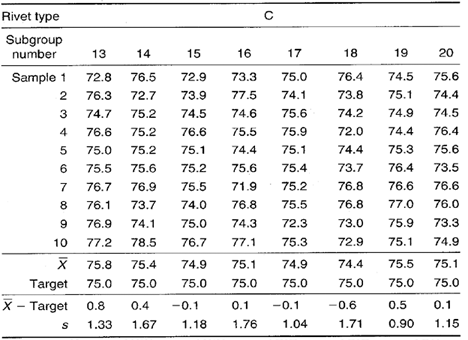## Target Xbar-s Chart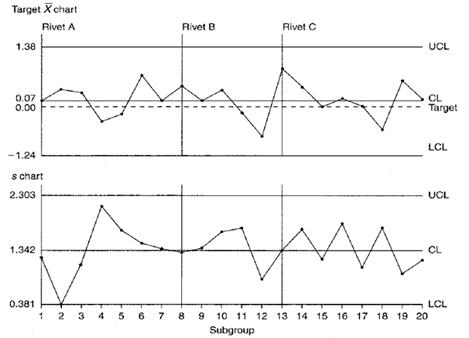Figure 2. Head height target Xbar-s control chart.

## Control Limit Calculations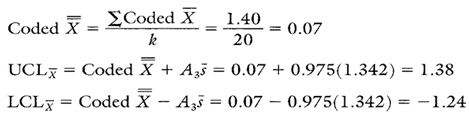Calculation 1. Calculations for target Xbar chart.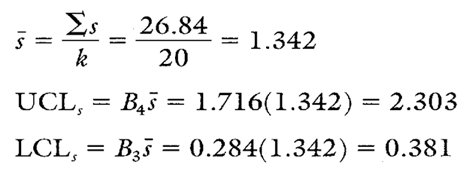Calculation 2. Calculations for s chart.

## Chart Interpretation

s chart: The chart is in control. This shows that the sample standard deviations of head heights for all three rivet types are similar.

Target Xbar chart: This chart is also in control. There are no indications of assignable causes. This means that the difference between the average head heights of all three rivet types and their respective targets is about the same.

## Recommendations

• Based on the target Xbar chart, the process is running very close to target regardless of rivet type. This is a situation where the process should not be adjusted.
• Even though the standard deviations are similar for all three rivet types, one will still need to calculate separate Cp and Cpk ratios. This is necessary because the engineering tolerances are different for each rivet type.

## Estimating the Process Average

Because the target Xbar chart is in control, the process average for all rivet types can be estimated using the coded X.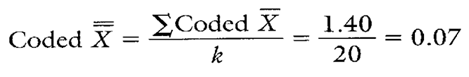Calculation 3. Estimate for the coded overall process average rivet head height (to be used in Cpk calculations for all three rivet types).

## Estimating Sigma

Because the s chart is in control, the process standard deviation can be estimated for all three rivet types using the formula found in Calculation 4.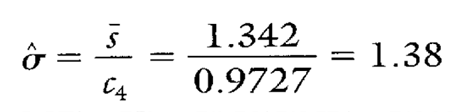Calculation 4. Estimating sigma using s.

## Calculating Process Capability and Performance Ratios

These ratios are calculated using coded data. The coded nominal for the head height characteristic is zero. Therefore, for rivet A, the coded USL is +10 and the coded LSL is –10. Following are calculations for the rivet A head height.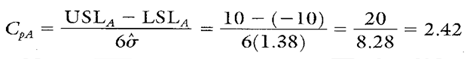Calculation 5. Cp calculation for rivet A head height.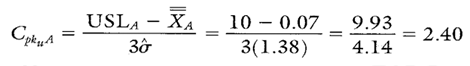Calculation 6. Cpk upper calculation for rivet A head height.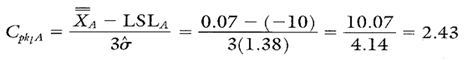Calculation 7. Cpk lower calculation for rivet A head height.

• Multiple parts or characteristics can be plotted on the same chart (provided they all exhibit similar variability).
• Data from gauges that are zeroed out on their target values can be plotted directly on the target Xbar without data coding or data transformation.
• Statistical control can be assessed for both the process and each unique part and/or characteristic being made in the process.
• Due to the large subgroup size, the Xbar chart is very sensitive to small process shifts.

• Requires software to efficiently handle the large amounts of data.
• The use of coded negative numbers can sometimes be confusing.
• When interpreting the target Xbar chart, both the zero line and the coded X must be taken into account. This accounts for some added complexity when interpreting the chart.

• Process capability and performance calculations for the B and C rivets are shown in Table 3.
• Because the target Xbar-s chart proved to be in control, the only values that change when calculating the capability ratios are the specification limits. The coded X and sigma values used to calculate Cp and Cpk ratios are the same for all three rivet types.

Table 3. Cp and Cpk calculations for B and C rivets.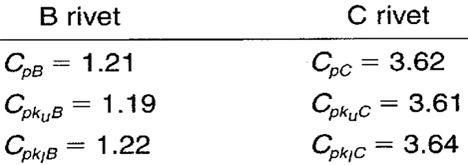When you use SPC software from InfinityQS, consuming the information provided by target Xbar-charts becomes faster and easier than ever. See how this type of analysis is surfaced in InfinityQS solutions.

FOOTNOTE:
1 Wise, Stephen A. and Douglas C. Fair. Innovative Control Charting: Practical SPC Solutions for Today’s Manufacturing Environment. Milwaukee, WI: ASQ Quality Press.

### Take the first step from quality to excellence

Take the Next Steps